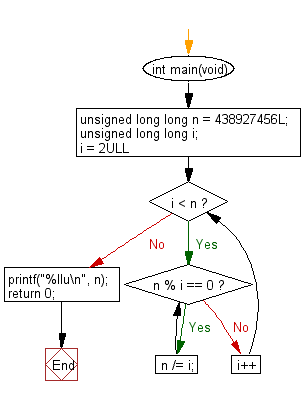﻿ C Program: Find largest prime factor of 438927456# C Exercises: Find the largest prime factor of the number 438927456

## C Programming Practice: Exercise-19 with Solution

The prime factors of 13195 are 5, 7, 13, 29.
Write a C programming to find the largest prime factor of the number 438927456?.

C Code:

``````#include <stdio.h>
int main(void)
{
unsigned long long n = 438927456L;
unsigned long long i;
for (i = 2ULL; i < n; i++) {
//1ULL is 'unsigned long long
while (n % i == 0) {
n /= i;
}
}
printf("%llu\n", n);
return 0;
}
``````

Sample Output:

```415651
```

Flowchart:## C Programming Code Editor:

What is the difficulty level of this exercise?

Test your Programming skills with w3resource's quiz.

﻿

## C Programming: Tips of the Day

Maximum value of int:

In C:

```#include <limits.h>
then use
int imin = INT_MIN; // minimum value
int imax = INT_MAX;```

or

```#include <float.h>

float fmin = FLT_MIN;  // minimum positive value
double dmin = DBL_MIN; // minimum positive value

float fmax = FLT_MAX;
double dmax = DBL_MAX;```

Ref : https://bit.ly/3fi8yk9